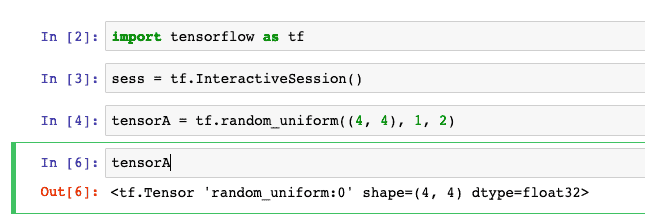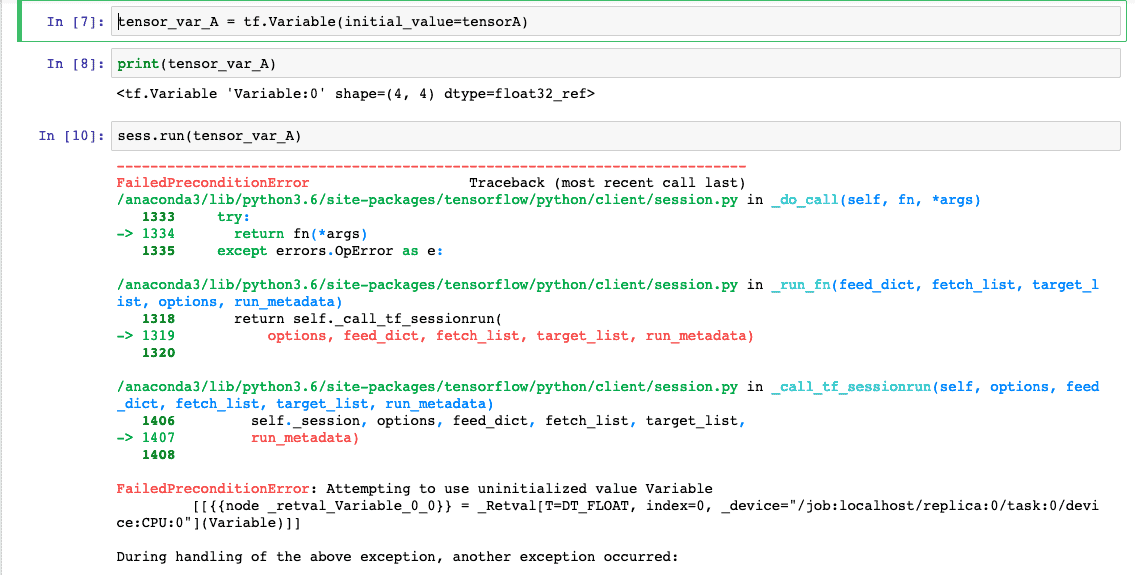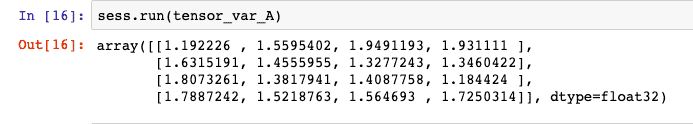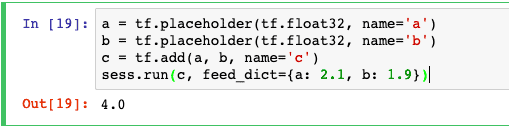1563590206

# TensorFlow Variables And Placeholders Tutorial With Example

TensorFlow Variables and Placeholders Tutorial With Example is today’s topic. TensorFlow is an open source machine learning framework developed by Google which can be used to the build neural networks and perform a variety of all machine learning tasks.

TensorFlow works on data flow graphs where nodes are the mathematical operations, and the edges are the data in the for tensors, hence the name Tensor-Flow.

Content Overview

• 1 Tensors
• 2 TensorFlow Variables
• 3 TensorFlow Placeholder
• 4 TensorFlow Variables and Placeholders Tutorial

## Tensors

A tensor is a central unit of data in TensorFlow. It consists of primitive values stored in the shape of a multidimensional array. The number of dimensions a tensor has is called its rank.

A rank 0 tensor is just a scalar. To keep things simple, we can say that a tensor in TensorFlow is instead a fancy name of an array and now we call dimension number as rank. One dimensional array or list is rank one tensor, and two-dimensional array or list is two rank tensor.

## TensorFlow Variables

When we train the model, we need to assign some weights and biases throughout the session.

TensorFlow variables can hold the values of biases and weights throughout the session.

You need to one thing keep in mind thatTensorFlow variables need to be initialized.

In TensorFlow variables are of great use when we are training models. As constants, we have to call a constructor to initialize a variable; the initial value can be passed in as an argument.

Variables can easily be added to the computational graph by calling a constructor.

## TensorFlow Placeholder

TensorFlow placeholders are initially empty and are used to feed in the actual training examples.

If we want to inject the data into a computation graph, we have to use the mechanism named as a placeholder. Placeholders are bound inside some expressions. The syntax of the placeholder is following.

``````placeholder(dtype, shape=None, name=None)

``````

Placeholders allow us to not to provide the data in advance for operations and computation graphs, and the data can be added in runtime from external sources as we train the Machine Learning models.

TensorFlow Placeholder does need to declare as a float32 datatype within an optional shape parameter.

## TensorFlow Variables and Placeholders Tutorial

Okay, we have covered enough theory, let’s see some practical example of TensorFlow Variables and Placeholders in Python Jupyter Notebook.

Also, you can find the basics of TesorFlow post.

Now, fire up the Jupyter Notebook and import the TensorFlow.

``````import tensorflow as tf

``````

You can run the cell by keyboard shortcut Ctrl + Enter.

In the next cell, we will write the following code.

``````sess = tf.InteractiveSession()

``````

The only difference with a regular Session is that an InteractiveSession installs itself as the default session on construction. We do not need to write that with** tf.Session() as sess** code whenever we need to perform some operations.

Once we run the above code, we do not need to start the session again for that Jupyter Notebook file.

Now, let’s define a random tensor using the following code.

``````tensorA = tf.random_uniform((4, 4), 1, 2)
tensorA

``````

Here, we have defined the **4*4 **matrix between the value 1 and 2. The values are random between 1 to 2.

When we try to display the tensorA, we will get the following output.Here, you can see that the datatype of tensorA is float32.

Now, in the next step, we will define a TensorFlow variable called tensor_var_A.

``````tensor_var_A = tf.Variable(initial_value=tensorA)

``````

Okay, now run the **tensor_var_A **variable.

``````sess.run(tensor_var_A)

``````

You will get an error like below.So, the error is saying that FailedPreconditionError: Attempting to use uninitialized value Variable.

That means, we need to first initialize the TensorFlow variable and then we can run that variable.

So, let’s do that first. Write the following code in the next cell.

``````init = tf.global_variables_initializer()

``````

Run the above cell and then write the following code in the next cell.

``````sess.run(init)

``````

Run the above cell, and all the variables are initialized. Now, we write that failed code again, and now you can see the 4*4 matrix.

``````sess.run(tensor_var_A)

``````

See the output below.Now, let’s create a TensorFlow Placeholder Example.

Define one placeholder using the following code in the next cell.

``````tfph = tf.placeholder(tf.float32, shape=(None, 5))

``````

The above code creates a TensorFlow placeholder, and its datatype is float32, and here **None **is the placeholder’s initial value of data. As time goes and our machine learning mode starts training, the data is filled in the placeholder. But, at the starting point, it is None.

We can use another example of TensorFlow Placeholder, which is the following code.

``````a = tf.placeholder(tf.float32, name='a')
b = tf.placeholder(tf.float32, name='b')
sess.run(c, feed_dict={a: 2.1, b: 1.9})

``````

Here, we have defined two placeholders and then create the third node to add both placeholders and run the operation. Remember, we are using Interactive Session. The output is following.So, this is how you can create TensorFlow Variables and Placeholders.

Finally, TensorFlow Variables and Placeholders Tutorial With Example is over.

#python #tensorflow #machine-learning